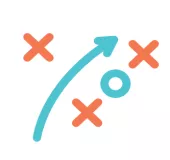# The demand for Writers and authors is projected to Grow 12% every year for the next 10 years, faster than any other career out there.

```!function(f,b,e,v,n,t,s) {if(f.fbq)return;n=f.fbq=function(){n.callMethod? n.callMethod.apply(n,arguments):n.queue.push(arguments)}; if(!f._fbq)f._fbq=n;n.push=n;n.loaded=!0;n.version='2.0'; n.queue=[];t=b.createElement(e);t.async=!0; t.src=v;s=b.getElementsByTagName(e); s.parentNode.insertBefore(t,s)}(window, document,'script', 'https://connect.facebook.net/en_US/fbevents.js%27); fbq('init', '674907722688658'); fbq('track', 'PageView');``` `` ```var _dcq = _dcq || []; var _dcs = _dcs || {}; _dcs.account = '4952312'; (function() { var dc = document.createElement('script'); dc.type = 'text/javascript'; dc.async = true; dc.src = '//tag.getdrip.com/4952312.js'; var s = document.getElementsByTagName('script'); s.parentNode.insertBefore(dc, s); })();```

There is no better time to
Learn, Practice, and Master writing than NOW!

## and I want to help you do that with this Course

This course was created with Newbie Filipinos in Mind.

Y﻿ou don't ﻿need ﻿any ﻿prior ﻿knowledge before starting this course

## In this Course, you Will Learn:

`(function(){var g=this;function h(b,d){var a=b.split("."),c=g;ain c||!c.execScript||c.execScript("var "+a);for(var e;a.length&&(e=a.shift());)a.length||void 0===d?c[e]?c=c[e]:c=c[e]={}:c[e]=d};function l(b){var d=b.length;if(0<d){for(var a=Array(d),c=0;c<d;c++)a[c]=b[c];return a}return[]};function m(b){var d=window;if(d.addEventListener)d.addEventListener("load",b,!1);else if(d.attachEvent)d.attachEvent("onload",b);else{var a=d.onload;d.onload=function(){b.call(this);a&&a.call(this)}}};var n;function p(b,d,a,c,e){this.h=b;this.j=d;this.l=a;this.f=e;this.g={height:window.innerHeight||document.documentElement.clientHeight||document.body.clientHeight,width:window.innerWidth||document.documentElement.clientWidth||document.body.clientWidth};this.i=c;this.b={};this.a=[];this.c={}}function q(b,d){var a,c,e=d.getAttribute("data-pagespeed-url-hash");if(a=e&&!(e in b.c))if(0>=d.offsetWidth&&0>=d.offsetHeight)a=!1;else{c=d.getBoundingClientRect();var f=document.body;a=c.top+("pageYOffset"in window?window.pageYOffset:(document.documentElement||f.parentNode||f).scrollTop);c=c.left+("pageXOffset"in window?window.pageXOffset:(document.documentElement||f.parentNode||f).scrollLeft);f=a.toString()+","+c;b.b.hasOwnProperty(f)?a=!1:(b.b[f]=!0,a=a<=b.g.height&&c<=b.g.width)}a&&(b.a.push(e),b.c[e]=!0)}p.prototype.checkImageForCriticality=function(b){b.getBoundingClientRect&&q(this,b)};h("pagespeed.CriticalImages.checkImageForCriticality",function(b){n.checkImageForCriticality(b)});h("pagespeed.CriticalImages.checkCriticalImages",function(){r(n)});function r(b){b.b={};for(var d=["IMG","INPUT"],a=[],c=0;c<d.length;++c)a=a.concat(l(document.getElementsByTagName(d[c])));if(0!=a.length&&a.getBoundingClientRect){for(c=0;d=a[c];++c)q(b,d);a="oh="+b.l;b.f&&(a+="&n="+b.f);if(d=0!=b.a.length)for(a+="&ci="+encodeURIComponent(b.a),c=1;c<b.a.length;++c){var e=","+encodeURIComponent(b.a[c]);131072>=a.length+e.length&&(a+=e)}b.i&&(e="&rd="+encodeURIComponent(JSON.stringify(t())),131072>=a.length+e.length&&(a+=e),d=!0);u=a;if(d){c=b.h;b=b.j;var f;if(window.XMLHttpRequest)f=new XMLHttpRequest;else if(window.ActiveXObject)try{f=new ActiveXObject("Msxml2.XMLHTTP")}catch(k){try{f=new ActiveXObject("Microsoft.XMLHTTP")}catch(v){}}f&&(f.open("POST",c+(-1==c.indexOf("?")?"?":"&")+"url="+encodeURIComponent(b)),f.setRequestHeader("Content-Type","application/x-www-form-urlencoded"),f.send(a))}}}function t(){var b={},d=document.getElementsByTagName("IMG");if(0==d.length)return{};var a=d;if(!("naturalWidth"in a&&"naturalHeight"in a))return{};for(var c=0;a=d[c];++c){var e=a.getAttribute("data-pagespeed-url-hash");e&&(!(e in b)&&0<a.width&&0<a.height&&0<a.naturalWidth&&0<a.naturalHeight||e in b&&a.width>=b[e].o&&a.height>=b[e].m)&&(b[e]={rw:a.width,rh:a.height,ow:a.naturalWidth,oh:a.naturalHeight})}return b}var u="";h("pagespeed.CriticalImages.getBeaconData",function(){return u});h("pagespeed.CriticalImages.Run",function(b,d,a,c,e,f){var k=new p(b,d,a,e,f);n=k;c&&m(function(){window.setTimeout(function(){r(k)},0)})});})();pagespeed.CriticalImages.Run('/ngx_pagespeed_beacon','https://www.wfhr.io/freelance-writing-for-beginners/?tve=true&tcbf=d42d386880','EX1L6AJvfC',true,false,'-vVcsY-uuuQ');``(function(){var g=this;function h(b,d){var a=b.split("."),c=g;ain c||!c.execScript||c.execScript("var "+a);for(var e;a.length&&(e=a.shift());)a.length||void 0===d?c[e]?c=c[e]:c=c[e]={}:c[e]=d};function l(b){var d=b.length;if(0<d){for(var a=Array(d),c=0;c<d;c++)a[c]=b[c];return a}return[]};function m(b){var d=window;if(d.addEventListener)d.addEventListener("load",b,!1);else if(d.attachEvent)d.attachEvent("onload",b);else{var a=d.onload;d.onload=function(){b.call(this);a&&a.call(this)}}};var n;function p(b,d,a,c,e){this.h=b;this.j=d;this.l=a;this.f=e;this.g={height:window.innerHeight||document.documentElement.clientHeight||document.body.clientHeight,width:window.innerWidth||document.documentElement.clientWidth||document.body.clientWidth};this.i=c;this.b={};this.a=[];this.c={}}function q(b,d){var a,c,e=d.getAttribute("data-pagespeed-url-hash");if(a=e&&!(e in b.c))if(0>=d.offsetWidth&&0>=d.offsetHeight)a=!1;else{c=d.getBoundingClientRect();var f=document.body;a=c.top+("pageYOffset"in window?window.pageYOffset:(document.documentElement||f.parentNode||f).scrollTop);c=c.left+("pageXOffset"in window?window.pageXOffset:(document.documentElement||f.parentNode||f).scrollLeft);f=a.toString()+","+c;b.b.hasOwnProperty(f)?a=!1:(b.b[f]=!0,a=a<=b.g.height&&c<=b.g.width)}a&&(b.a.push(e),b.c[e]=!0)}p.prototype.checkImageForCriticality=function(b){b.getBoundingClientRect&&q(this,b)};h("pagespeed.CriticalImages.checkImageForCriticality",function(b){n.checkImageForCriticality(b)});h("pagespeed.CriticalImages.checkCriticalImages",function(){r(n)});function r(b){b.b={};for(var d=["IMG","INPUT"],a=[],c=0;c<d.length;++c)a=a.concat(l(document.getElementsByTagName(d[c])));if(0!=a.length&&a.getBoundingClientRect){for(c=0;d=a[c];++c)q(b,d);a="oh="+b.l;b.f&&(a+="&n="+b.f);if(d=0!=b.a.length)for(a+="&ci="+encodeURIComponent(b.a),c=1;c<b.a.length;++c){var e=","+encodeURIComponent(b.a[c]);131072>=a.length+e.length&&(a+=e)}b.i&&(e="&rd="+encodeURIComponent(JSON.stringify(t())),131072>=a.length+e.length&&(a+=e),d=!0);u=a;if(d){c=b.h;b=b.j;var f;if(window.XMLHttpRequest)f=new XMLHttpRequest;else if(window.ActiveXObject)try{f=new ActiveXObject("Msxml2.XMLHTTP")}catch(k){try{f=new ActiveXObject("Microsoft.XMLHTTP")}catch(v){}}f&&(f.open("POST",c+(-1==c.indexOf("?")?"?":"&")+"url="+encodeURIComponent(b)),f.setRequestHeader("Content-Type","application/x-www-form-urlencoded"),f.send(a))}}}function t(){var b={},d=document.getElementsByTagName("IMG");if(0==d.length)return{};var a=d;if(!("naturalWidth"in a&&"naturalHeight"in a))return{};for(var c=0;a=d[c];++c){var e=a.getAttribute("data-pagespeed-url-hash");e&&(!(e in b)&&0<a.width&&0<a.height&&0<a.naturalWidth&&0<a.naturalHeight||e in b&&a.width>=b[e].o&&a.height>=b[e].m)&&(b[e]={rw:a.width,rh:a.height,ow:a.naturalWidth,oh:a.naturalHeight})}return b}var u="";h("pagespeed.CriticalImages.getBeaconData",function(){return u});h("pagespeed.CriticalImages.Run",function(b,d,a,c,e,f){var k=new p(b,d,a,e,f);n=k;c&&m(function(){window.setTimeout(function(){r(k)},0)})});})();pagespeed.CriticalImages.Run('/ngx_pagespeed_beacon','https://www.wfhr.io/freelance-writing-for-beginners/?tve=true&tcbf=158eca5938','EX1L6AJvfC',true,false,'JdCmnAKo8fs');`###### Proper research techniques to write accurate articles###### Basic Sales Copy to increase client sales###### Different kinds of Blog posts###### Overcome Writers block and write effectively###### Write like a Native to be able to charge higher rates###### Build A Portfolio to WOW your clients###### Find jobs inside Upwork and Outside Upwork

PLUS! You Will Also Get These BONUSES:An SEO Writing Checklist for you to ensure proper SEO is being followed in your postsAmerican Idiom Worksheet that you can utilize when writing for AmericansA Formatting Checklist to make sure your content looks great to clients and audiences

## What People are Saying...#### GLEN FOUND THE COURSES EFFECTIVE AND ACTIONABLE - AND THEY BROUGHT HIM RESULTS!

I would just to thank Jason Dulay for his courses. The lessons were very effective. After following all the actions steps that were given through the course, I’ve been getting invitations at a high rate. After two months of applying on upwork using what I learned from Jason’s courses, I successfully landed: 2 hourly Gigs and 2 Fixed Price Projects!

##### Glen Gallarde (Web Designer)#### BERNICE FOUND THE LESSONS EASY TO UNDERSTAND, EVEN FOR TODDLERS!

Jason's course is encouraging, practical and real. I get relevant and specific advice as regards starting a home based career. Videos are short without sacrificing content (good for someone like me who has the attention span of a 2 year old). Masustansya, baga. And best of all, accessible si Jason. He is sincere and approachable. Just started pero may mga leads na ako. Given time and as my skills improve, I know ill hit my goal income.

##### 60-Day ﻿﻿Money ﻿﻿Back Guarantee!

Enroll in this course for 60-days and if you find that you haven't learned anything valuable, just let me know and I will refund your money 100% Guaranteed!

~ Jason Dulay

## What are you waiting for? Enroll Today!

### Enroll in Freelance Writing for Beginners• Start Your Career As a Writer
• Learn different kinds of content that you can write